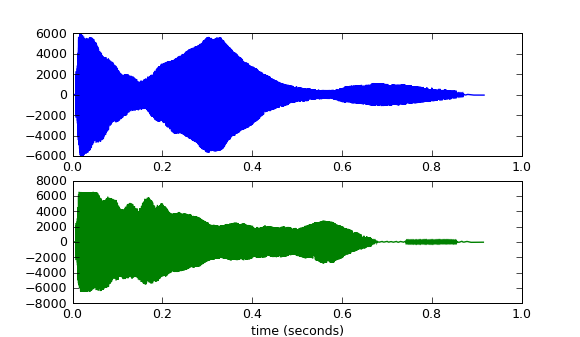# 声音的输入输出

## 读写Wave文件

WAV是Microsoft开发的一种声音文件格式，虽然它支持多种压缩格式，不过它通常被用来保存未压缩的声音数据（PCM脉冲编码调制)。WAV有三个重要的参数：声道数、取样频率和量化位数。

• 声道数：可以是单声道或者是双声道
• 采样频率：一秒内对声音信号的采集次数，常用的有8kHz, 16kHz, 32kHz, 48kHz, 11.025kHz, 22.05kHz, 44.1kHz
• 量化位数：用多少bit表达一次采样所采集的数据，通常有8bit、16bit、24bit和32bit等几种

### 读Wave文件

``````# -*- coding: utf-8 -*-
import wave
import pylab as pl
import numpy as np

# 打开WAV文档
f = wave.open(r"c:\WINDOWS\Media\ding.wav", "rb")

# 读取格式信息
# (nchannels, sampwidth, framerate, nframes, comptype, compname)
params = f.getparams()
nchannels, sampwidth, framerate, nframes = params[:4]

# 读取波形数据
f.close()

#将波形数据转换为数组
wave_data = np.fromstring(str_data, dtype=np.short)
wave_data.shape = -1, 2
wave_data = wave_data.T
time = np.arange(0, nframes) * (1.0 / framerate)

# 绘制波形
pl.subplot(211)
pl.plot(time, wave_data)
pl.subplot(212)
pl.plot(time, wave_data, c="g")
pl.xlabel("time (seconds)")
pl.show()
``````WindowsXP的经典"叮"声的波形

``````import wave
f = wave.open(r"c:\WINDOWS\Media\ding.wav", "rb")
``````

• getparams：一次性返回所有的WAV文件的格式信息，它返回的是一个组元(tuple)：声道数, 量化位数（byte单位）, 采样频率, 采样点数, 压缩类型, 压缩类型的描述。wave模块只支持非压缩的数据，因此可以忽略最后两个信息：

``````params = f.getparams()
nchannels, sampwidth, framerate, nframes = params[:4]
``````
• getnchannels, getsampwidth, getframerate, getnframes等方法可以单独返回WAV文件的特定的信息。

``````str_data = f.readframes(nframes)
``````

``````wave_data = np.fromstring(str_data, dtype=np.short)
``````

``````wave_data.shape = -1, 2
``````

``````wave_data = wave_data.T
``````

``````time = np.arange(0, nframes) * (1.0 / framerate)
``````

### 写Wave文件

``````# -*- coding: utf-8 -*-
import wave
import numpy as np
import scipy.signal as signal

framerate = 44100
time = 10

# 产生10秒44.1kHz的100Hz - 1kHz的频率扫描波
t = np.arange(0, time, 1.0/framerate)
wave_data = signal.chirp(t, 100, time, 1000, method='linear') * 10000
wave_data = wave_data.astype(np.short)

# 打开WAV文档
f = wave.open(r"sweep.wav", "wb")

# 配置声道数、量化位数和取样频率
f.setnchannels(1)
f.setsampwidth(2)
f.setframerate(framerate)
# 将wav_data转换为二进制数据写入文件
f.writeframes(wave_data.tostring())
f.close()
``````

10-12行通过调用scipy.signal库中的chrip函数，产生长度为10秒、取样频率为44.1kHz、100Hz到1kHz的频率扫描波。由于chrip函数返回的数组为float64型，需要调用数组的astype方法将其转换为short型。

18-20行分别设置输出WAV文件的声道数、量化位数和取样频率，当然也可以调用文件对象的setparams方法一次性配置所有的参数。最后21行调用文件的writeframes方法，将数组的内部的二进制数据写入文件。writeframes方法会自动的更新WAV文件头中的长度信息(nframes)，保证其和真正的数据数量一致。

## 用pyAudio播放和录音

pyAudio是开源声音库PortAudio( http://www.portaudio.com )的Python绑定，目前它只支持阻塞式的输入输出模式。所谓阻塞式就是需要用户的程序主动地去读写输入输出流。虽然阻塞式在功能上有所局限，但是由于编程比较简单，非常适合一些处理声音的脚本程序开发。

### 播放

``````# -*- coding: utf-8 -*-
import pyaudio
import wave

chunk = 1024

wf = wave.open(r"c:\WINDOWS\Media\ding.wav", 'rb')

p = pyaudio.PyAudio()

# 打开声音输出流
stream = p.open(format = p.get_format_from_width(wf.getsampwidth()),
channels = wf.getnchannels(),
rate = wf.getframerate(),
output = True)

# 写声音输出流进行播放
while True:
if data == "": break
stream.write(data)

stream.close()
p.terminate()
``````

PyAudio类的open函数有许多参数：

• rate - 取样频率
• channels - 声道数
• format - 取样值的量化格式 (paFloat32, paInt32, paInt24, paInt16, paInt8 ...)。在上面的例子中，使用get_format_from_width方法将wf.sampwidth()的返回值2转换为paInt16
• input - 输入流标志，如果为True的话则开启输入流
• output - 输出流标志，如果为True的话则开启输出流
• input_device_index - 输入流所使用的设备的编号，如果不指定的话，则使用系统的缺省设备
• output_device_index - 输出流所使用的设备的编号，如果不指定的话，则使用系统的缺省设备
• frames_per_buffer - 底层的缓存的块的大小，底层的缓存由N个同样大小的块组成
• start - 指定是否立即开启输入输出流，缺省值为True

### 录音

``````# -*- coding: utf-8 -*-
from pyaudio import PyAudio, paInt16
import numpy as np
from datetime import datetime
import wave

# 将data中的数据保存到名为filename的WAV文件中
def save_wave_file(filename, data):
wf = wave.open(filename, 'wb')
wf.setnchannels(1)
wf.setsampwidth(2)
wf.setframerate(SAMPLING_RATE)
wf.writeframes("".join(data))
wf.close()

NUM_SAMPLES = 2000      # pyAudio内部缓存的块的大小
SAMPLING_RATE = 8000    # 取样频率
LEVEL = 1500            # 声音保存的阈值
COUNT_NUM = 20          # NUM_SAMPLES个取样之内出现COUNT_NUM个大于LEVEL的取样则记录声音
SAVE_LENGTH = 8         # 声音记录的最小长度：SAVE_LENGTH * NUM_SAMPLES 个取样

# 开启声音输入
pa = PyAudio()
stream = pa.open(format=paInt16, channels=1, rate=SAMPLING_RATE, input=True,
frames_per_buffer=NUM_SAMPLES)

save_count = 0
save_buffer = []

while True:
# 读入NUM_SAMPLES个取样
# 将读入的数据转换为数组
audio_data = np.fromstring(string_audio_data, dtype=np.short)
# 计算大于LEVEL的取样的个数
large_sample_count = np.sum( audio_data > LEVEL )
print np.max(audio_data)
# 如果个数大于COUNT_NUM，则至少保存SAVE_LENGTH个块
if large_sample_count > COUNT_NUM:
save_count = SAVE_LENGTH
else:
save_count -= 1

if save_count < 0:
save_count = 0

if save_count > 0:
# 将要保存的数据存放到save_buffer中
save_buffer.append( string_audio_data )
else:
# 将save_buffer中的数据写入WAV文件，WAV文件的文件名是保存的时刻
if len(save_buffer) > 0:
filename = datetime.now().strftime("%Y-%m-%d_%H_%M_%S") + ".wav"
save_wave_file(filename, save_buffer)
save_buffer = []
print filename, "saved"
``````